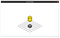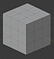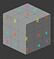# Build an isometric 3D game in 2D — #2 Texture render order

This is part 2 of the 2d/3d(game/engine) conversion used in my puzzle game: Ladder Box, which is available on Steam:

# Problem

In the first article, we know that we must create new blocks in order, otherwise it’ll have some weird render like this:weird render

# Render Order

Imagine you are looking at a Rubik’s Cube like sojust made this in Blenderlayer order
`“1” : V3(3,3,3) => 3+3+3 = 9 [ z_index = 9]“2” : V3(2,3,3,)/V3(3,2,3)/V3(3,3,2) => 2+3+3 = 8 [ z_index = 8 ]and the rest goes on`

# Write the code

First, let’s also calculate z_index in our GridUtils.game_to_engine:

`func game_to_engine(x:int,y:int,z:int) -> Vector3: var _rtn_2d = Vector2(0,0) _rtn_2d += x*SINGLE_X _rtn_2d += z*SINGLE_Z _rtn_2d += y*SINGLE_Y var _z = x+y+z return Vector3(_rtn_2d.x,_rtn_2d.y,_z)`
`onready var SINGLE_Y = Vector2(0,-texture_h/2) * TEXTURE_SCALE`
`class_name BlockBaseextends Spritevar game_pos:Vector3func _ready(): passfunc set_game_pos(x:int,y:int,z:int): game_pos = Vector3(x,y,z) var engine_pos = Grid.game_to_engine(x,y,z) self.position = Vector2(engine_pos.x,engine_pos.y) self.z_index = engine_pos.z passfunc set_game_posv3(new_pos:Vector3): set_game_pos(int(new_pos.x),int(new_pos.y),int(new_pos.z)) pass`
`extends BlockBasefunc _ready(): pass`
`# scripts/main.gdextends Node2Dconst movable = preload("res://scenes/movable.tscn")const unmovable = preload("res://scenes/unmovable.tscn")onready var grid_texture = load("res://textures/grid.png")func _ready(): for x in range(6):  for z in range(6):   \$floor_tile.set_cell(x,z,0)   pass # you can add blocks however you want ,but might got something weird. new_movable(0,0,0) new_movable(0,1,0) new_unmovable(3,0,3) passfunc new_movable(x,y,z): var _m = movable.instance() \$movable.add_child(_m) _m.set_game_pos(x,y,z) passfunc new_unmovable(x,y,z): var _u = unmovable.instance() \$unmovable.add_child(_u) _u.set_game_pos(x,y,z) pass`
• use function from block_base to set position

# Run the scene

now if we run the scene:

`new_movable(0,1,0)new_movable(0,0,0)`